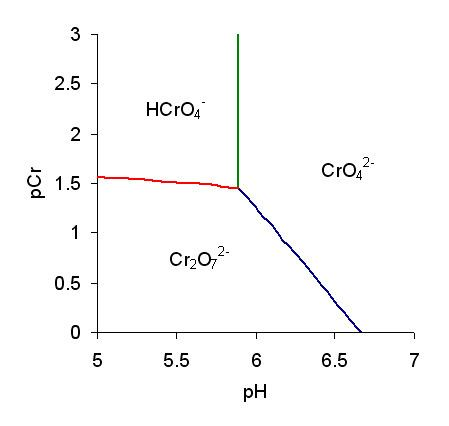# Predominance diagram

Updated on
Covid-19A predominance diagram purports to show the conditions of concentration and pH where a chemical species has the highest concentration in solutions in which there are multiple acid-base equilibria. The lines on a predominance diagram indicate where adjacent species have the same concentration. Either side of such a line one species or the other predominates, that is, has higher concentration relative to the other species.

To illustrate a predominance diagram, part of the one for chromate is shown at the right. pCr stands for minus the logarithm of the chromium concentration and pH stands for minus the logarithm of the hydrogen ion concentration. There are two independent equilibria, with equilibrium constants defined as follows.

A third equilibrium constant can be derived from K1 and KD.

The species H2CrO4 and HCr
2
O
7
are only formed at very low pH so they do not appear on this diagram. Published values for log K1 and log KD are 5.89 and 2.05, respectively. Using these values and the equality conditions, the concentrations of the three species, chromate CrO2−
4
, hydrogen chromate HCrO
4
and dichromate Cr
2
O2−
7
can be calculated, for various values of pH, by means of the equilibrium expressions. The chromium concentration is calculated as the sum of the species' concentrations in terms of chromium content.

[ Cr ] = [ CrO 4 2 ] + [ HCrO 4 ] + 2 [ Cr 2 O 7 2 ] ; pCr = log 10 [ Cr ]

The three species all have concentrations equal to 1/KD at pH = pK1, for which [Cr] = 4/KD. The three lines on this diagram meet at that point.

1. Green line. Chromate and hydrogen chromate have equal concentrations. Setting [CrO2−
4
] equal to [HCrO
4
] in eq. 1, [H+] = 1/K1, or pH = log K1. This relationship is independent of pCr, so it requires a vertical line to be drawn on the predominance diagram.
2. Red line. Hydrogen chromate and dichromate have equal concentrations. Setting [HCrO
4
] equal to [Cr
2
O2−
7
] in Eq. 2, [HCrO
4
] = 1/KD; from Eq. 1, then, [CrO2−
4
] = 1/K1[H+].
3. Blue line. Chromate and dichromate have equal concentrations. Setting [CrO2−
4
] equal to [Cr
2
O2−
7
] in Eq. 3 gives [CrO2−
4
] = 1/β2[H+]2.

The predominance diagram is interpreted as follows. The chromate ion is the predominant species in the region to the right of the green and blue lines. Above pH ~6.75 it is always the predominant species. At pH < 5.89 (pH < pK1) the hydrogen chromate ion is predominant in dilute solution but the dichromate ion is predominant in more concentrated solutions.

Predominance diagrams can become very complicated when many polymeric species can be formed as, for example, with vanadate, molybdate and tungstate. Another complication is that many of the higher polymers are formed extremely slowly, such that equilibrium may not be attained even in months, leading to possible errors in the equilibrium constants and the predominance diagram.

Topics